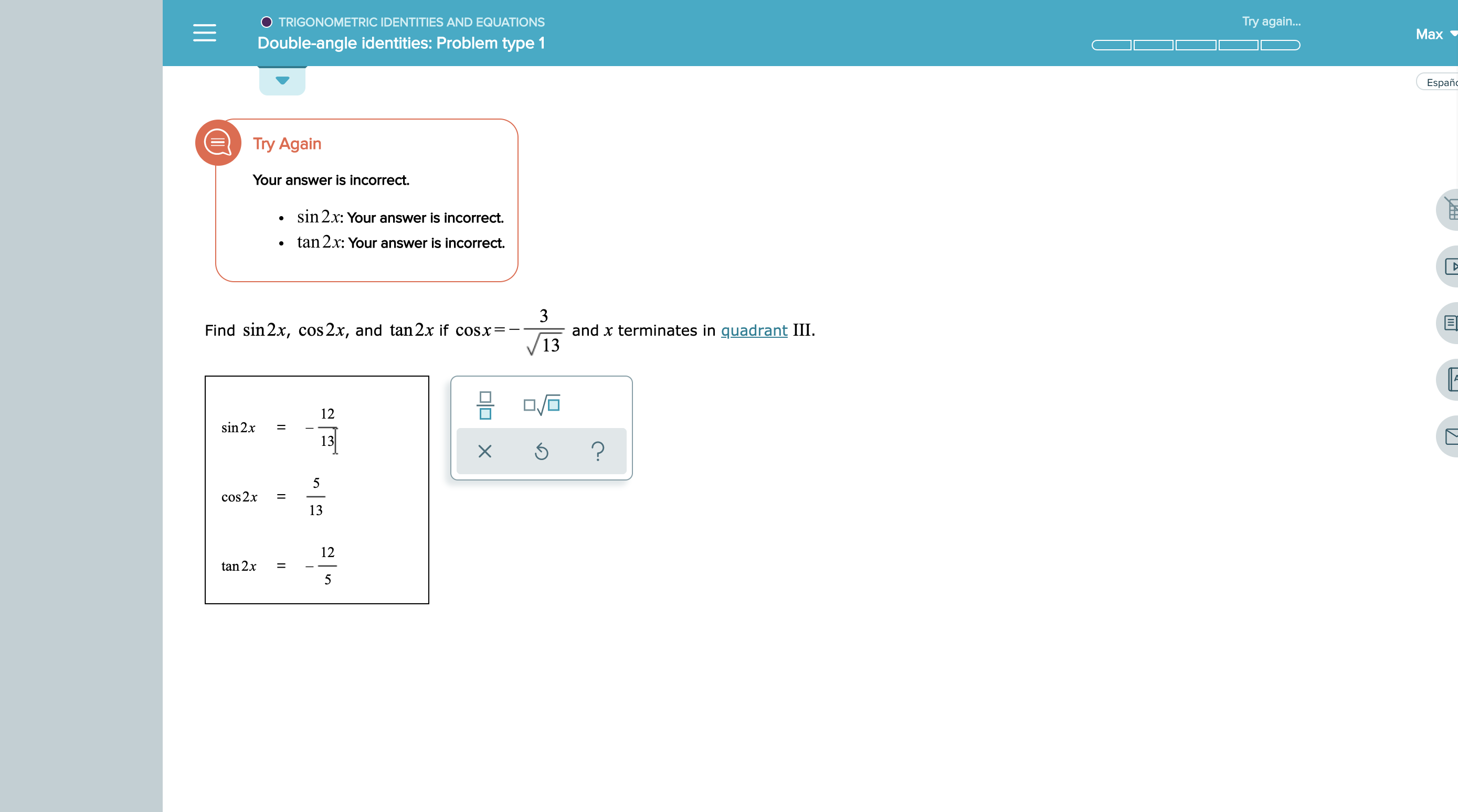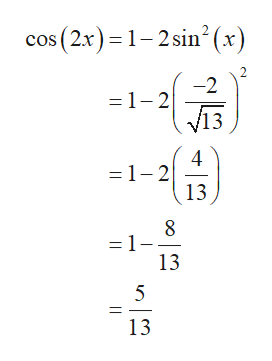# Try again...TRIGONOMETRIC IDENTITIES AND EQUATIONSMaxDouble-angle identities: Problem type 1EspanTry AgainYour answer is incorrectsin 2x: Your answer is incorrect.tan 2x: Your answer is incorrect.3and x terminates in quadrant III13Find sin 2x, cos 2x, and tan2x if cosx=12sin 2x=13?X5cos2x1312tan 2x5

Question
267 views

See attachedhelp_outlineImage TranscriptioncloseTry again... TRIGONOMETRIC IDENTITIES AND EQUATIONS Max Double-angle identities: Problem type 1 Espan Try Again Your answer is incorrect sin 2x: Your answer is incorrect. tan 2x: Your answer is incorrect. 3 and x terminates in quadrant III 13 Find sin 2x, cos 2x, and tan2x if cosx= 12 sin 2x = 13 ? X 5 cos2x 13 12 tan 2x 5 fullscreen
check_circle

Step 1

Calculate the value for sinx and tanx where Cosx=adjacent/hypotenuse.

Step 2

Apply the formula for sin(2x) and substitute the value of sin(x) and cos(x).

Step 3

Apply the formula for cos(2x) and substitut...help_outlineImage Transcriptionclosecos (2x) 1-2 sin2(x) 2 =1-2 v13 4 =1-2 13 8 =1- 13 13 fullscreen

### Want to see the full answer?

See Solution

#### Want to see this answer and more?

Solutions are written by subject experts who are available 24/7. Questions are typically answered within 1 hour.*

See Solution
*Response times may vary by subject and question.
Tagged in

### Trigonometric Ratios